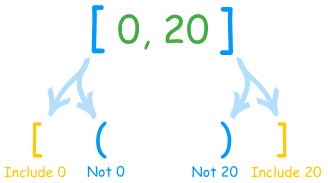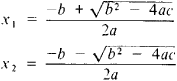# Category Archives: URI Online Judge

## URI Online Judge | 1030 Flavious Josephus Legend

This is level 3 problem so will do it later. But this video will help for  sure.

## URI Online Judge Solution | 1049 Animal

strcmp in c/c++ issues is the hard part nothing else…Just try to learn this 🙂
Logical condition based problem it was.

## URI Online Judge Solution | 1048 Salary Increase

My failed code:
http://ideone.com/4o96LX

My succeeded accepted code:

## URI Online Judge Solution | 1332 One-Two-Three | UVA 12289

Editorial:

Here we have to think like a child :D….So first we took a character array where we will take input string.And if the input is = 5 then it will print three as per the input set.And then when we enter the value input like ‘t’ and ‘o’ and ‘w’ then we can make it out from the string.So we have added this like this if t and w or if t or o or w or o is available then we will print 2 in the output where in string array t in the position 1, w is in the position 2, o is in the position 3

So basically the output case 2 we made the condition in the logic.

Note: In logic we must have to follow the sequence of the text One,Two,Three as suppose in two it can be two,too,ooo,owo  and as the input will do at most 100 words.So this steps has been taken 🙂

## URI Online Judge Solution| 1168 LED

https://raw.githubusercontent.com/gmendonca/uri-problem-solutions/master/1168.cpp

## URI Online Judge | 1042 Simple Sort

https://www.urionlinejudge.com.br/judge/en/problems/view/1042

http://www.mathsisfun.com/definitions/ascending-order.html
and
http://www.mathsteacher.com.au/year7/ch02_power/06_asc/asc.htm

## URI Online Judge Solution | 1041 Coordinates of a Point

If x!=0 and y==0 then it will be in the y asix and
If y!=0 and x==0 then it will be in the x axis

that means you have to understand if what the value is equivalent to 0 then it will be in that axis

And one thing = means assignment operator if x=y then if x=5 then y=5

and == means comparison operator if x==y then if x==4 and y==5 then x!=y so it will be false

and if x=5 and y=5 then x==y and it is true

Reference:
http://www.mathsisfun.com/data/cartesian-coordinates.html

code:

and like this can do

Here in this code you have to think about the flow which normally starts from the 0 and the compiler starts punctuating the code from Zero 0.

logic flow can be start from 0 then can be happen through greater than or equal

It’s better to do in C/C++ main format

## URI Online Judge Solution | 1040 – Average 3

Keep check on the line “Calculate the average with weights 2,3,4 e 1” actually that means you have to use the avergae formula like this

n1*2+n2*3+n3*4+n4*1/(2+3+4+1)

and check this line “followed by the typed score”..You have to input this value before showing

Note: URI,UVA,codeforces and in various online judges there is presentation error if you dont give new line after every output value that means in C you have to give “\n” in every printf/output line otherwise it will show “Presentation Error” on the OJ’s

## URI Online Judge Solution | 1040 – Average 3

Keep check on the line “Calculate the average with weights 2,3,4 e 1” actually that means you have to use the avergae formula like this

n1*2+n2*3+n3*4+n4*1/(2+3+4+1)

and check this line “followed by the typed score”..You have to input this value before showing

Note: URI,UVA,codeforces and in various online judges there is presentation error if you dont give new line after every output value that means in C you have to give “\n” in every printf/output line otherwise it will show “Presentation Error” on the OJ’s

## URI Online Judge Solution | 1040 – Average 3

Keep check on the line “Calculate the average with weights 2,3,4 e 1” actually that means you have to use the avergae formula like this

n1*2+n2*3+n3*4+n4*1/(2+3+4+1)

and check this line “followed by the typed score”..You have to input this value before showing

Note: URI,UVA,codeforces and in various online judges there is presentation error if you dont give new line after every output value that means in C you have to give “\n” in every printf/output line otherwise it will show “Presentation Error” on the OJ’s

## URI Online Judge Solution | 1040 – Average 3

Keep check on the line “Calculate the average with weights 2,3,4 e 1” actually that means you have to use the avergae formula like this

n1*2+n2*3+n3*4+n4*1/(2+3+4+1)

and check this line “followed by the typed score”..You have to input this value before showing

Note: URI,UVA,codeforces and in various online judges there is presentation error if you dont give new line after every output value that means in C you have to give “\n” in every printf/output line otherwise it will show “Presentation Error” on the OJ’s

## URI Online Judge Solution |1038 Snack

Here I tried to write everything in standard C++

## To Carry or not to Carry

Dont forget to use format specifier for unsigned int %u

## URI Online Judge Solution | 1037 Interval

http://www.mathsisfun.com/sets/intervals.html

Check there about the interval notation section…

## Interval Notation

In “Interval Notation” we just write the beginning and ending numbers of the interval, and use:

• [ ] a square bracket when we want to include the end value, or
• ( ) a round bracket when we don’t

Like this:### Example: (5, 12]

Means from 5 to 12, do not include 5, but do include 12

## URI Online Judge Solution| 1036 Bhaskara’s Formula

An equation ax2 + bx + c = 0 where a,b and c are any number  and called the coefficient of the equation
It is known as quadratic equation because any seconbd degree polynomial equation is known as quadratic equation.This equation has two roots x1 and x2This is Bhaskara’s Formula or Bhaskaracharya’s Formula where discriminant D or Del = b24ac
If D>0 then root of quadratic equation are real and unequal
If D=0 then root of quadratic equation are real and equal
If D<0 then root of quadratic equation are conjugate complex number